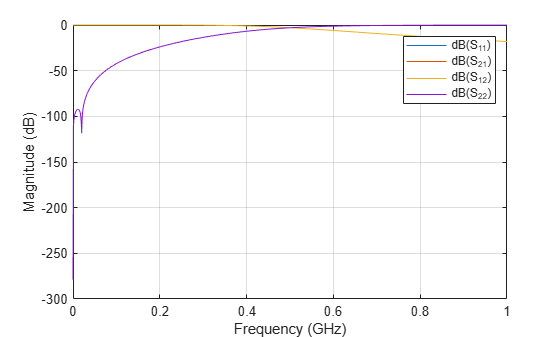Documentation

## Description

`lcladder` class creates an LC ladder object that you can add to an existing circuit. Create filters and calculate s-parameters of filters using `lcladder` class. You can also add the `lcladder` object to an existing circuit.

You can also use the `lcladder` object to convert the `rffilter` object to an lcladder ```LCLad = lacladdder(rffiltobj)``` where `rffilterobj` is an `rffilter` object.## Creation

### Syntax

``lcobj = lcladder(topology,inductances,capacitances)``
``lcobj = lcladder(rffilterobj)``
``lcobj = lcladder(___,lcname)``

### Description

example

````lcobj = lcladder(topology,inductances,capacitances)` creates an LC ladder object, `lcobj`, with a topology, `top`, inductor values, `l`, and capacitor values, `c`.```
````lcobj = lcladder(rffilterobj)` creates an LC ladder object, `lcobj`, with a name, `lcname`from an RF filter object, `rffilter`. ```
````lcobj = lcladder(___,lcname)` creates an LC ladder object, `lcobj`, with a name, `lcname`. `lcname` must be a character vector.```

## Properties

expand all

Topology type of the LC ladder, specified as a one of the following character vector:

• `'lowpasspi'`: Low-pass pi filter

• `'lowpasstee'`: Low-pass tee filter

• `'highpasspi'`: High-pass pi filter

• `'highpasstee'`: High-pass tee filter

• `'bandpasspi'`: Band-pass pi filter

• `'bandpasstee'`: Band-pass tee filter

• `'bandstoppi'`: Band-stop pi filter

• `'bandstoptee'`: Band-stop tee filter

Set the topology type in the `topology` argument of the syntax.

Example: `'lowpasspi'`

Inductor values in LC ladder, specified as a numeric scalar or vector in henries. Set the inductor value in the `inductances` argument of the syntax.

Example: `3.18e-8`

Capacitor values in LC ladder, specified as a numeric scalar or vector in farads. Set the capacitor value in the `c` argument of the syntax.

Example: `[6.37e-12 6.37e-12]`

Name of LC ladder object, specified as a character vector. Set the name of the LC ladder in `lcname` argument of the syntax.

Example: `'lcfilter'`

Number of ports in LC ladder object. specified as a scalar. This value is always `2`.

Terminal names of LC ladder object, specified as the cell vector, `{'p1+' 'p2+' 'p1-' 'p2-'}`. An LC ladder object always has four terminals: two terminals associated with the first port (`'p1+'` and `'p1-'`) and two terminals associated with the second port (`'p2+'` and `'p2-'`).

Example: `{'p1+' 'p2+' 'p1-' 'p2-'}`

Parent circuit nodes connected to LC ladder object terminals, specified as a vector of integers. This property appears only after the LC ladder object is added to a circuit.

### Note

"ParentNodes" are only displayed after the capacitor has been added

into a circuit.

Full path of the circuit to which the LC ladder object belongs, specified as character vector. This path appears only after the inductor is added to the circuit.

### Note

"ParentPath" is only displayed after the capacitor has been added

into a circuit.

## Examples

collapse all

Create a low-pass pi LC ladder object with inductor value of 3.18e-8 and capacitor value of 6.37e-12. Calculate and plot the s-parameters.

```L = 3.18e-8; C = [6.37e-12 6.37e-12]; lpp = lcladder('lowpasspi',L,C)```
```lpp = lcladder: LC Ladder element Topology: 'lowpasspi' Inductances: 3.1800e-08 Capacitances: [6.3700e-12 6.3700e-12] Name: 'lcfilt' NumPorts: 2 Terminals: {'p1+' 'p2+' 'p1-' 'p2-'} ```
``` freq = 0:1e6:1e9; S = sparameters(lpp,freq); rfplot(S)```